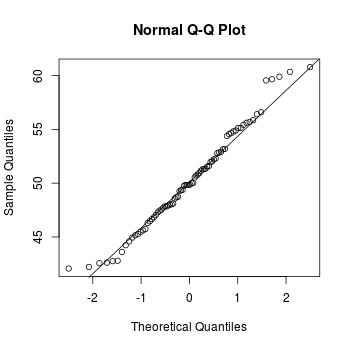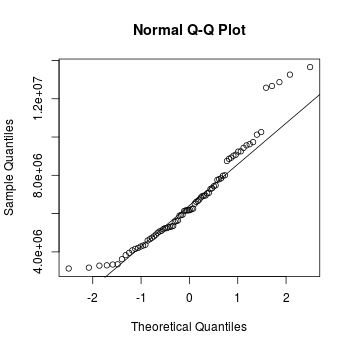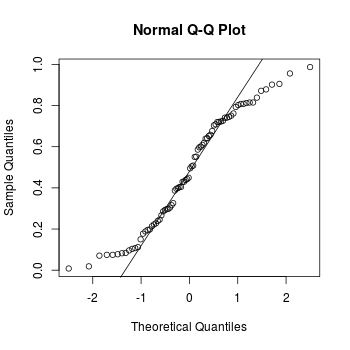## Problem

You want to compare the distribution of your data to another distribution. This is often used to check whether a sample follows a normal distribution, to check whether two samples are drawn from the same distribution.

## Solution

``````set.seed(183)
# Normally distributed numbers
x <- rnorm(80, mean=50, sd=5)

# Uniformly distributed numbers
z <- runif(80)
``````
``````# Compare the numbers sampled with rnorm() against normal distribution
qqnorm(x)
qqline(x)

# The same numbers to the 4th power, compared to the normal distribution
qqnorm(x^4)
qqline(x^4)

# Numbers sampled from the flat distribution, compared to normal
qqnorm(z)
qqline(z)
``````